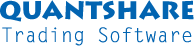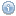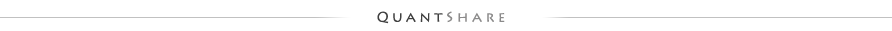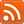#twitter-follow { border: 0;position: fixed; top: 240px; left:0;} #live-support { border: 0;position: fixed; top: 370px; left:0;} #knowledge-base { border: 0;position: fixed; top: 170px; left:0;}

## Mean and Standard deviation - Z-Score

by QuantShare, 5038 days agoThis composite calculates daily standard deviation of one-bar rate of return for all securities. It also calculates the average one-bar rate of return for all securities. The average or mean value is stored in the "volume" field; it is multiplied by 10000 because the "volume" field accepts only integer values and so that we do not lose the precision of the number. The daily standard deviation is stored in the "Close" field.

This item allows you to calculate the standard score or the z-score value of any security or stock using a simple formula. The standard score is used to measure the number of standard deviations a value is above or below the mean.

For the one-bar rate of return indicator, the z-score value of a particular asset for a particular bar is the value of the one-bar rate of return for that bar minus the average value calculated by the composite and stored in the "volume" field then divided by the standard deviation value that is stored in the "close" field.

The QuantShare formula is (The composite name is "_RETURN SDV")
a = (roc(close, 1) - (Ticker("_RETURN SDV",volume,LastData)/10000)) / Ticker("_RETURN SDV",close,LastData);

In this item as well as in the example, I have used the one-bar rate of return, but you could use any function, indicator or time-series. You just need to update the composite item and change the variable named "function". Currently, this variable is set to "roc(close, 1)", but you can change it to "roc(close, 10)" for example to calculate the 10-bar rate of return, or "rsi(close, 14)" to calculate the Relative Strength Index.
If you use "rsi(close, 14)" in the composite formula:
a = (rsi(close, 14) - (Ticker("_RETURN SDV",volume,LastData)/10000)) / Ticker("_RETURN SDV",close,LastData);

The following line calculates the z-score of the Relative Strength Index for all the security' bars. A z-score value of one means that the Relative Strength Index (RSI) of the security is one standard deviation above the mean of the RSI for all securities.

To have a shorter formula, you can download the z-score function I have uploaded here: Z-Score (Standard Score); It uses the same formula as the one described above.

Note that I have also used two filters in the composite formula. The first filter cap the value of the rate of return at 100% while the second is used to reject or ignore symbols whose share price is below two.

What is this?

 Type: Composite Index Object ID: 381 Country: All Market: All Style: Technical Analysis

 Related objectsEmpty
Number of reviews
Average rate
Click to rate this item
Number of rates the current object received
Report an object
if you can't run it for example or if it contains errors
Click to report this object

 Random Blog Posts Trend Following and Moving Averages Put-Call Ratio for Individual Stocks Historical Market Data What's new in this trading software? Historical volatility estimators Short Selling Stocks Stock split & dividend Survivorship bias Show All

Number of reviews
Average rate
Click to rate this item
Number of rates the current object received
Report an object
if you can't run it for example or if it contains errors
Click to report this objectSupport Contact Us Trading Forum How-to Lessons Manual Company About Us Privacy Terms of Use Copyright © 2023 QuantShare.comTrading financial instruments, including foreign exchange on margin, carries a high level of risk and is not suitable for all investors. The high degree of leverage can work against you as well as for you. Before deciding to invest in financial instruments or foreign exchange you should carefully consider your investment objectives, level of experience, and risk appetite. The possibility exists that you could sustain a loss of some or all of your initial investment and therefore you should not invest money that you cannot afford to lose. You should be aware of all the risks associated with trading and seek advice from an independent financial advisor if you have any doubts.